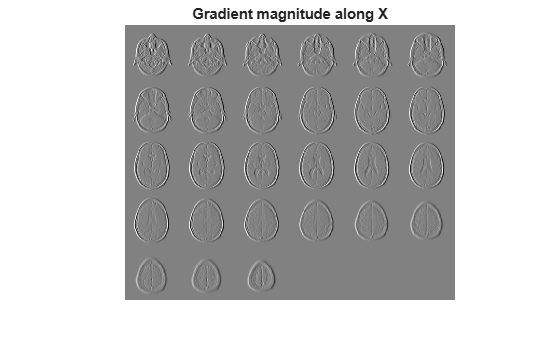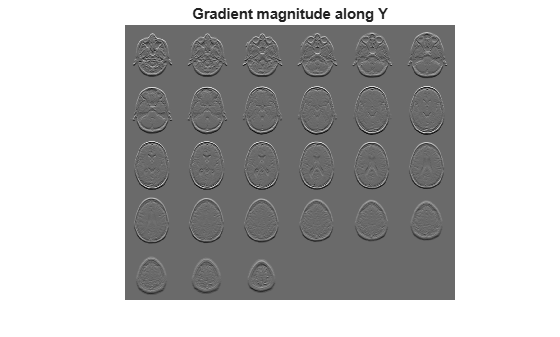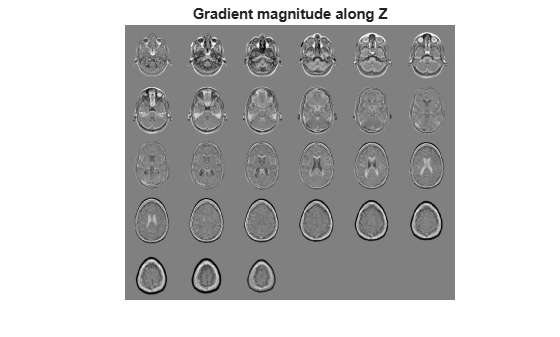Main Content

# imgradientxyz

Find directional gradients of 3-D image

## Syntax

``````[Gx,Gy,Gz] = imgradientxyz(I)``````
``````[Gx,Gy,Gz] = imgradientxyz(I,method)``````

## Description

example

``````[Gx,Gy,Gz] = imgradientxyz(I)``` returns the directional gradients `Gx`, `Gy`, and `Gz` of the 3-D grayscale or binary image `I`.```
``````[Gx,Gy,Gz] = imgradientxyz(I,method)``` calculates the directional gradients using the specified `method`.```

## Examples

collapse all

Read 3-D data and prepare it for processing.

```volData = load('mri'); sz = volData.siz; vol = squeeze(volData.D);```

Calculate the directional gradients.

`[Gx, Gy, Gz] = imgradientxyz(vol);`

Visualize the directional gradients as a montage.

```figure, montage(reshape(Gx,sz(1),sz(2),1,sz(3)),'DisplayRange',[]) title('Gradient magnitude along X')`````` figure, montage(reshape(Gy,sz(1),sz(2),1,sz(3)),'DisplayRange',[]) title('Gradient magnitude along Y')`````` figure, montage(reshape(Gz,sz(1),sz(2),1,sz(3)),'DisplayRange',[]) title('Gradient magnitude along Z')```## Input Arguments

collapse all

Input image, specified as a 3-D grayscale image or 3-D binary image.

Data Types: `single` | `double` | `int8` | `int16` | `int32` | `int64` | `uint8` | `uint16` | `uint32` | `uint64` | `logical`

Gradient operator, specified as one of the following values.

Value

Meaning

```'sobel' ```

Sobel gradient operator. The gradient of a pixel is a weighted sum of pixels in the 3-by-3-by-3 neighborhood. For example, in the depth (z) direction, the weights in the three planes are:

plane `z-1`plane `z`plane `z+1`
```[ 1 3 1 3 6 3 1 3 1 ] ```
```[ 0 0 0 0 0 0 0 0 0 ] ```
```[ -1 -3 -1 -3 -6 -3 -1 -3 -1 ] ```

`'prewitt'`

Prewitt gradient operator. The gradient of a pixel is a weighted sum of pixels in the 3-by-3-by-3 neighborhood. For example, in the depth (z) direction, the weights in the three planes are:

plane `z-1`plane `z`plane `z+1`
```[ 1 1 1 1 1 1 1 1 1 ] ```
```[ 0 0 0 0 0 0 0 0 0 ] ```
```[ -1 -1 -1 -1 -1 -1 -1 -1 -1 ] ```

`'central' `

Central difference gradient. The gradient of a pixel is a weighted difference of neighboring pixels. For example, in the depth (z) direction, ```dI/dz = (I(z+1) - I(z-1))/2```.

`'intermediate'`

Intermediate difference gradient. The gradient of a pixel is the difference between an adjacent pixel and the current pixel. For example, in the depth (z) direction, ```dI/dz = I(z+1) - I(z)```.

When applying the gradient operator at the boundaries of the image, `imgradientxyz` assumes values outside the bounds of the image are equal to the nearest image border value. This behavior is similar to the `'replicate'` boundary option in `imfilter`.

Data Types: `char` | `string`

## Output Arguments

collapse all

Horizontal gradient, returned as a numeric matrix of the same size as image `I`. The horizontal (x) axis points in the direction of increasing column subscripts. `Gx` is of class `double`, unless the input image `I` is of class `single`, in which case `Gx` is of class `single`.

Data Types: `single` | `double`

Vertical gradient, returned as a numeric matrix of the same size as image `I`. The vertical (y) axis points in the direction of increasing row subscripts. `Gy` is of class `double`, unless the input image `I` is of class `single`, in which case `Gy` is of class `single`.

Data Types: `single` | `double`

Depth gradient, returned as a 3-D numeric array of the same size as image `I`. The depth (z) axis points in the direction of increasing plane subscripts. `Gz` is of class `double`, unless the input image `I` is of class `single`, in which case `Gz` is of class `single`.

## Algorithms

`imgradientxyz` does not normalize the gradient output. If the range of the gradient output image has to match the range of the input image, consider normalizing the gradient image, depending on the `method` argument used. For example, with a Sobel kernel, the normalization factor is 1/44, for Prewitt, the normalization factor is 1/18.

## See Also

Introduced in R2016a

Download ebook